# Important 2 Marks Questions for CBSE 12th Chemistry

Class 12 chemistry important 2 mark questions with answers are provided here. These important questions are based on CBSE board curriculum and correspond to the most recent Class 12 chemistry syllabus. By practising these Class 12 important 2 mark questions, students will be able to quickly review all of the ideas covered in the chapter and prepare for the Class 12 Annual examinations as well as other entrance exams such as NEET and JEE.

Download Class 12 Chemistry Important 2 Mark Questions with Answers PDF by clicking on the button below.

## Chemistry Sample Paper Analysis## Class 12 Chemistry Important 2 Mark Questions with Answers

Question 1:

An aqueous solution of sodium chloride freezes below 273 K. Explain the lowering in freezing points of water with the help of a suitable diagram.

When NaCl is dissolved in water, the vapour pressure of the solution decreases, and at lower temperatures, the vapour pressures of the solid and liquid solvents become equal, resulting in a decrease in freezing point.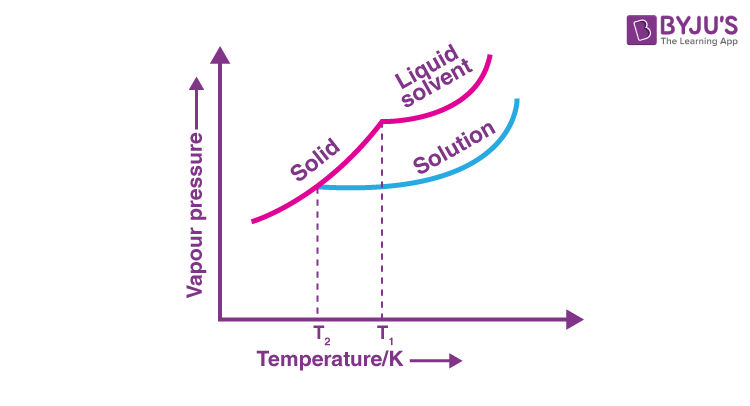Question 2:

The conductivity of 0.001 M acetic acid is 4 x 10-5 S/cm. Calculate the dissociation constant of acetic acid, if molar conductivity at infinite dilution for acetic acid is 390 S cm2/mol.

C = 0.001 M, k = 4 ✕ 10–5 s/cm

$$\begin{array}{l}Λm =\frac{1000\times k}{C}\end{array}$$
$$\begin{array}{l}Λm = \frac{1000\times 4\times 10^{-5}}{0.001}\end{array}$$

Λm = 40 S cm2 mol-1

Λ° = 390 S cm2 mol–1

ɑ = degree of dissociation of CH3COOH

$$\begin{array}{l}\alpha =\frac{\lambda _{m}}{\lambda ^{\circ }}=\frac{40}{390}=0.1025\approx 0.103\end{array}$$
$$\begin{array}{l}K_{a}=\frac{\left [ CH_{3}COO^{-} \right ]\left [ H^{+} \right ]}{\left [ CH_{3}COOH \right ]}\end{array}$$
$$\begin{array}{l}K_{a}= \frac{C\alpha \times C\alpha }{C\left ( 1-\alpha \right )}=\frac{C\alpha ^{2}}{C\left ( 1-\alpha \right )}\end{array}$$
$$\begin{array}{l}K_{a} =\frac{0.001\times \left ( 0.103 \right )^{2}}{1-0.103}\end{array}$$

Ka = 1.18 ✕10–5

Question 3:

The standard electrode potential for the Daniell cell is 1.1 V. Calculate the standard Gibbs energy for the cell reaction. (F = 96500 C mol-1)

The net cell reaction in Daniel cell is-

Zn (s) + Cu2+(aq) → Cu (s) + Zn2+(aq)

Therefore, the value of n=2

∆G°= -nFE°cell

∆G°= -2×96500 C mol-1×1.1 V

∆G°= – 212300 CV = -212300J [∵Coloumb × Volt = Joule]

∆G°= -212.3 kJ

Question 4:

Explain the following:

1. Same substance can act both as colloids and crystalloids

Yes, the same substance can act as colloids and crystalloids because both of these states are related to the particle size of the substance. The substance will behave as a crystalloid if it is less than 1 nm in size. If it is greater than 1000 nm, it will behave as a colloid in the solution.

1. Artificial rain is caused by spraying salt over clouds.

By spraying salt over clouds, the colloidal water particles in the clouds are neutralised and coagulated into larger water drops, resulting in artificial rain.

Question 5:

How are the following colloidal solutions prepared?

1. Sulphur in water

Sulphur sol is made by oxidising H2S with SO2. It can be understood by the following reaction-

SO2 + 2H2S → 3S + 2H2O

1. Gold in water

Gold sol is made by reducing AuCl3 with formaldehyde. The equation for the following reaction is-

2AuCl3 + 3HCHO + 3H2O → 2Au (sol) + 3HCOOH + 6HCl

Question 6:

Give reasons for the following

1. Alumina is dissolved in cryolite for electrolysis instead of being electrolyzed directly.

Cryolite serves two purposes in alumina electrolysis.

(a) It lowers the melting point of the mixture to around 1250 degrees Celsius.

(b) It improves the melt’s electrical conductivity.

1. Zinc oxide can be reduced to metal by heating with carbon but Cr2O3 cannot be reduced by heating with carbon.

Because carbon has a higher affinity for oxygen than zinc, oxides of moderately reactive metals such as Zn can be reduced by carbon, resulting in ZnO being reduced to Zn and carbon combining with oxygen to form CO. Whereas chromium oxides cannot be reduced by carbon because carbon has a lower affinity for oxygen than chromium, due to which chromium oxide cannot be reduced by carbon.

Question 7:

Explain the following:

1. NO2 readily forms a dimer.

This is due to the fact that NO2 has an odd number of valence electrons. Dimerisation converts it to a stable N2O4 molecule with an even number of electrons.

1. BiCl3 is more stable than BiCl5.

Due to the inert pair effect, the stability of the +5 oxidation state decreases in group-15 while the stability of the +3 oxidation state increases. As a result, BiCl3 is more stable than BiCl5.

Question 8:

What is Lanthanoid contraction? What are its two consequences?

Lanthanide contraction refers to the steady decrease in the size of lanthanide ions as their atomic number increases.

Consequences:

• There is no regular trend in the group’s ionisation potential and
• There is a similarity of the second and third transition groups

Question 9:

Explain the following:

1. Gabriel Phthalimide reaction

The Gabriel synthesis is a chemical reaction that transforms primary alkyl halides into primary amines. Traditionally, the reaction uses potassium phthalimide. The name of the reaction comes from the German chemist Siegmund Gabriel. The reaction has been generalized for applications in the alkylation of sulfonamides and imides & their deprotection in order to obtain amines. Alkylation of ammonia is quite inefficient, therefore, it is substituted with phthalimide anion in the Gabriel synthesis.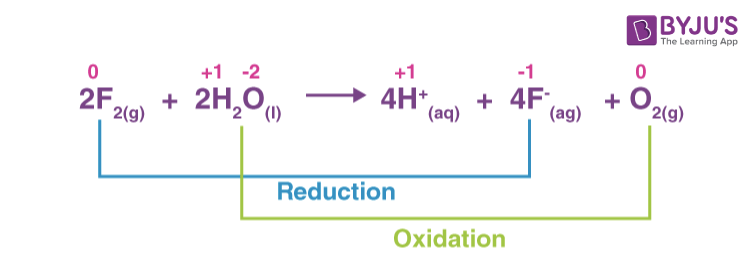1. Coupling reaction

The coupling reaction occurs when diazonium salt reacts with phenols and aromatic amines to form azo compounds with the general formula Ar−N=N−Ar. The coupling with phenol occurs in a mildly alkaline medium.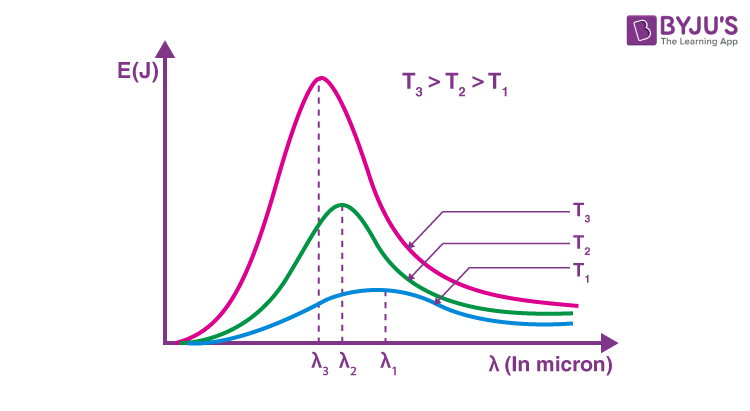Question 10:

Give a medical test to distinguish between the following pairs of compounds.

1. Aniline and Ethylamine

Ethylamine and aniline can be distinguished using the azo-dye test. A dye is obtained when aromatic amines react with HNO2 (NaNO2 + dil.HCl) at 0-5°C, followed by a reaction with the alkaline solution of 2-naphthol. The dye is usually yellow, red, or orange in colour. Aliphatic amines give a brisk effervescence due (to the evolution of N2 gas) under similar conditions.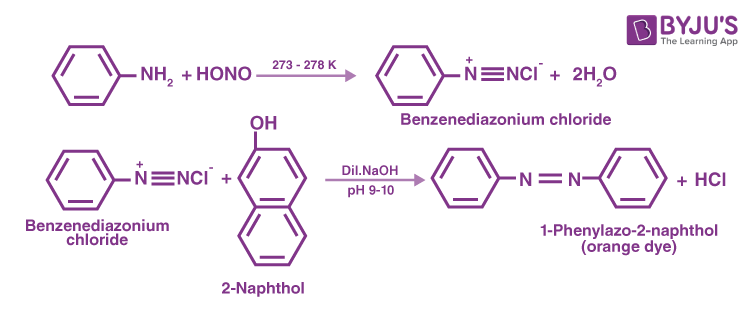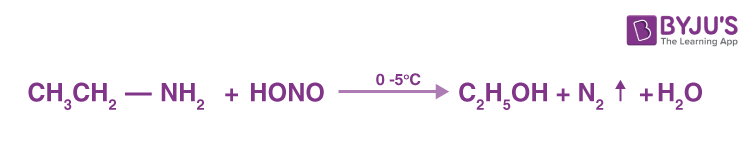1. Ethylamine and Dimethylamine

When heated with chloroform and potassium hydroxide solution, ethylamine produces a foul-smelling ethyl isocyanide. This test is not performed by dimethylamine.

C2H5NH2 + CHCl3 + 3KOH → C2H5NC + 3KCl + 3H2O

Question 11:

Explain the following terms with suitable examples:

1. Cationic detergents

Cationic detergents are quaternary ammonium salts of chlorides, bromides or acetates. A cationic component of these detergents consists of a long hydrocarbon chain and the nitrogen atom has a positive charge in it.

Examples: Cetyltrimethylammonium bromide is a popular cationic detergent and is used in hair conditioners.

1. Anionic detergents

Anionic detergent is a synthetic detergent in which a lipophilic hydrocarbon group of the molecule is an anion. A detergent molecule consists of a long hydrocarbon chain and a water-soluble negative ionic group i.e sodium salts of the long-chain sulfonated alcohols or hydrocarbons.

Example: Sodium alkyl sulphates and Sodium alkyl benzene sulphonates

Question 12:

An element occurs in bcc structure. It has a cell edge length of 250 pm. Calculate the molar mass if its density is 8.0 g cm-3. Also, calculate the radius of an atom of this element.

a = 250 pm = 250 ×10-10 cm, d = 8g cm-3, z = 2 (for bcc), M=?

$$\begin{array}{l}8=\frac{2\times M}{\left ( 250\times 10^{-3} \right )^{3}\times \left ( 6.02\times 10^{23} \right )}\end{array}$$

M=

$$\begin{array}{l}\frac{\left ( 250\times 10^{-3} \right )^{3}\times \left ( 6.022\times 10^{23} \right )\times 8}{2}\end{array}$$
$$\begin{array}{l}M=\frac{9.409\times 8}{2}=37.64 g mol^{-1}\end{array}$$

For bcc unit cell,

4r=√3 a

$$\begin{array}{l}r=\frac{\sqrt{3}a}{4}=\frac{1.732\times 250}{4}\end{array}$$

∴r=108.25 pm

Question 13:

Calculate the number of unit cells in 8·1 g of aluminium if it crystallizes in a face-centred cubic (f.c.c.) structure. (Atomic mass of Al = 27 g mol-1)

Given that, Mass of aluminium w = 8.1g

Number of atoms of Al,

$$\begin{array}{l}N_{Al}=\frac{mass}{Molar mass}\times N_{A}=\frac{w}{M}\times N_{A}=\frac{8.1}{27}\times N_{A}\end{array}$$

As it crystallises in a face-centred cubic (f.c.c.) structure, each unit cell contains four atoms.

Therefore, number of unit cells=

$$\begin{array}{l}\frac{8.1\times 6.02\times 10^{23}}{27\times 4}=4.52\times 10^{22} unit cells\end{array}$$

Question 14:

1. Arrange the following compounds in the increasing order of their acid strength:
p-cresol, p-nitrophenol, phenol

The nitro group in p-nitrophenol exhibits -M effect, which stabilises the conjugate anion, making it the most acidic.

The methyl group in p-cresol exhibits a +H effect, which destabilises the conjugate anion and causes it to be the least acidic.

As a result, the acidity order will be:

p-cresol < phenol < p-nitrophenol

1. Write the mechanism (using curved arrow notation) of the following reaction:
$$\begin{array}{l}CH_2=CH_2 \overset{H_3O^+}{\rightarrow} CH_3-CH^+_2+H_2O\end{array}$$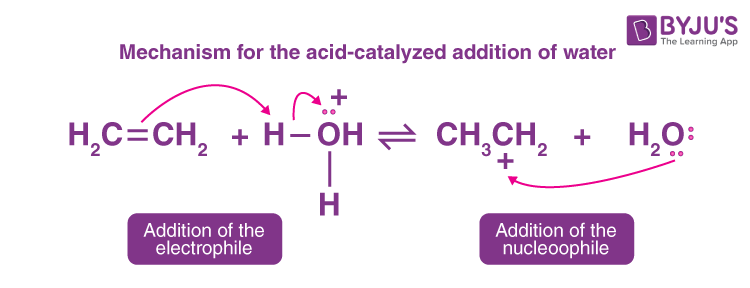Question 15:

Write the structures of the products when Butan-2-ol reacts with the following :

1. CrO3

$$\begin{array}{l}CH_{3}CH_{2}CHOHCH_{3}\xrightarrow[]{CrO_{3}}CH_{3}CH_{2}COCH_{3}\end{array}$$
1. SOCl2

$$\begin{array}{l}CH_{3}CH_{2}CHOHCH_{3}\xrightarrow[]{SOCl_{2}}CH_{3}CH_{2}CHClCH_{3}\end{array}$$

Question 16:

Draw the structures of the following :

1. H2SO3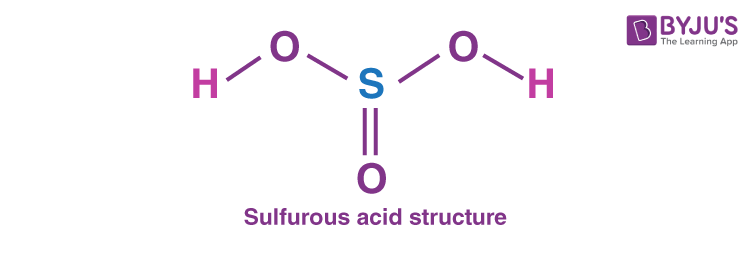1. HClO3Question 17:

Write the name of the cell which is generally used in hearing aids. Write the reactions taking place at the anode and the cathode of this cell.

The cell which is used in hearing aids is the Mercury cell. The reactions taking place are as follows-

At anode: Zn(Hg) + 2OH→ ZnO (s) + H2O + 2e

At Cathode: HgO + H2O + 2e → Hg (l) + 2OH

Question 18:

Using IUPAC norms write the formula for the following :

(a) Sodium dicyanidoaurate(I)

Na[Au(CN)2]

(b) Tetraamminechloridonitrito-N-platinum(IV) sulphate

[Pt(NH3)4Cl(NO2)]SO4

Question 19:

Write the name of the cell which is generally used in hearing aids. Write the reactions taking place at the anode and the cathode of this cell.

The cell which is used in hearing aids is the Mercury cell. The reactions taking place are as follows-

At anode: Zn(Hg) + 2OH→ ZnO (s) + H2O + 2e

At Cathode: HgO + H2O + 2e → Hg (l) + 2OH

Question 20:

Using IUPAC norms write the formulae for the following : [1 + 1 = 2]

1. Sodium dicyanidoaurate (I)

Na[Au(CN)2]

1. Tetraamminechloridonitrito-N-plantinum(IV) sulphate

[Pt(NH3)4Cl(NO2)]SO4

Question 21:

(i) Write the colligative property which is used to find the molecular mass of macromolecules.

Osmotic Pressure is the colligative property that is used to find the molecular mass of macromolecules.

(ii) In non-ideal solution, what type of deviation shows the formation of minimum boiling azeotropes?

In a non-ideal solution, positive deviation shows the formation of minimum boiling azeotropes.

Question 22:

Write the structures of the following:

(i) (HPO3)3

Answer.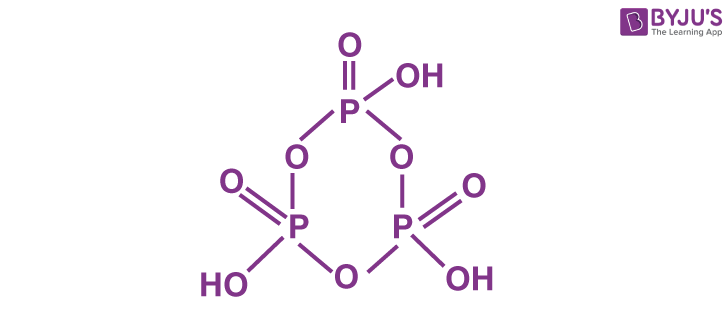(ii) XeF4Question 23:

When a coordination compound NiCl2 · 6H2O is mixed with AgNO3, 2 moles of AgCl are precipitated per mole of the compound. Write:

(i) Structural formula of the complex

The structural formula of the complex compound is- [Cr(H2O)5Cl]Cl2.H2O

(ii) IUPAC name of the complex

The IUPAC name of the complex is Pentaaquachlorochromium(III)dichloride monohydrate

Question 24:

For a reaction: 2NH3(g)

$$\begin{array}{l}\overset{Pt}{\rightarrow}\end{array}$$
N2(g) + 3H2(g) Rate = k

(i) Write the order and molecularity of this reaction.

The order of the reaction is zero and the molecularity of the reaction is two.

(ii) Write the unit of k.

The unit of k is

$$\begin{array}{l} mol L^{-1} t^{-1}\end{array}$$

Question 25:

Write the chemical equations involved in the following reactions:

(i) Kolbe’s reaction

When phenol is treated with sodium hydroxide, the ion phenoxide is formed. The phenoxide ion produced is more reactive than phenol in electrophilic aromatic substitution reactions. As a result, it undergoes an electrophilic substitution reaction with carbon dioxide, a weak electrophile. The primary product is ortho-hydroxybenzoic acid (salicylic acid). This is commonly referred to as Kolbe’s reaction.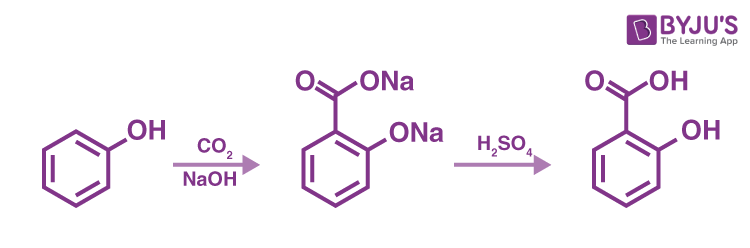(ii) Friedal-Crafts acetylation of anisole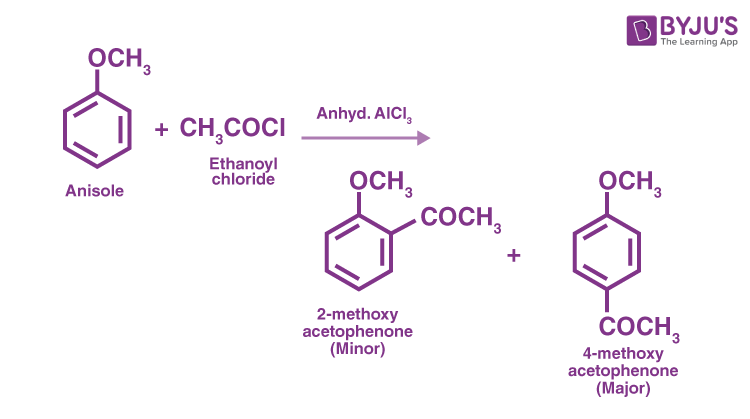Question 26:

How do you convert:

(i) Phenol to toluene

When phenol is distilled with zinc dust, the corresponding aromatic hydrocarbons are produced. The benzene is then treated with CH3Cl to produce toluene.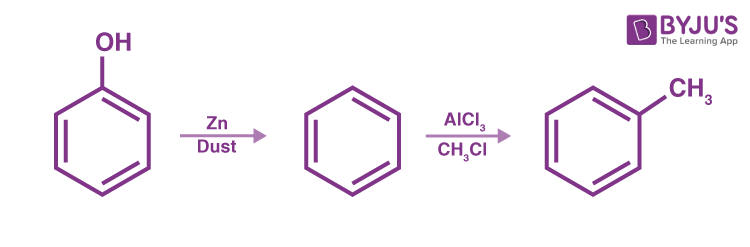(ii) Formaldehyde to Ethanol

After treating formaldehyde with magnesium methyl bromide, the product obtained will be hydrolyzed to yield ethanol.

$$\begin{array}{l}HCHO+CH_{3}MgBr\to CH_{3}CH_{2}\left ( O-Mg-Br \right )\xrightarrow[H_{2}O]{H^{^{+}},}CH_{3}CH_{2}OH+ HO-Mg-Br\end{array}$$

Question 27:

Why do transition elements show variable oxidation states? How is the variability in oxidation states of d-block different from that of the p-block elements?

The energies of (n-1)d orbitals and ns orbitals are nearly identical in transition elements. As a result, electrons from both can participate in bond formation, resulting in variable oxidation states.

In transition elements, the oxidation states differ from each other by unity. For e.g., Fe+2 and Fe+3, Cu+ and Cu2+, etc., whereas in p-block elements, the oxidation states differ by units of two, e.g., Sn2+ and Sn3+, Pb2+ and Pb4+, etc.

Higher oxidation states in transition elements are more stable for heavier elements in a group. For e.g., Mo (VI) and W (VI) are more stable than Cr (VI) in group 6, whereas lower oxidation states in p-block elements are more stable for heavier elements due to the inert pair effect, e.g., Pb(Il) is more stable than Pb(IV) in group 16

Question 28:

(i) Write down the IUPAC name of the following complex : [Pt(NH3)(H2O)Cl2]

The IUPAC name is Triamine aqua dichloro platinum (II) complex.

(ii) Write the formula for the following complex : tris(ethane-1,2-diamine)chromium(III) chloride

The formula is [Cr(en)3]Cl3.

Question 29:

Calculate the time to deposit 1.5 g of silver at cathode when a current of 1.5 A was passed through the solution of AgNO3. (Molar mass of Ag = 108 g mol-1, 1 F = 96500 C mol-1)

At cathode: Ag+ + e → Ag

Q = nF

Q = 1 ✕ 96500 C

Since, 108 g of silver is deposited by passing 96500 C

∴ 1.5 g of silver is deposited by passing =

$$\begin{array}{l}\frac{96500}{108 g}\times 1.5 g = 1340.27 C\end{array}$$

Q = 1340.27 C

Q = It

$$\begin{array}{l}t= \frac{Q}{I}= \frac{1340.27 C}{1.5 A} = 893.51 sec\end{array}$$

∴ t = 14.85 minutes

Question 30:

Write the reagents used in the following reaction-

CH3–COOH

$$\begin{array}{l}\overset{?}{\rightarrow}\end{array}$$
CH3–COCl

By reacting with phosphorus pentachloride, carboxylic acid is converted to acid chloride. Therefore, the reagent is PCl5

Question 31:

Arrange the following compounds in increasing order of their property as indicated

1. CH3CHO, C6H5CHO, HCHO (reactivity towards nucleophilic addition reaction)

A nucleophilic addition reaction occurs when a chemical compound with an electron-deficient or electrophilic double or triple bond, a π bond, reacts with an electron-rich reactant, known as a nucleophile, resulting in the disappearance of the double bond and the formation of two new single, or σ. Therefore, C6H5CHO < CH3CHO < HCHO

1. 2,4-dinitro benzoic acid, 4-methoxy benzoic acid, 4-nitrobenzoic acid (acidic character)

The acidity of the oxide increases as the central atom becomes more electronegative. The electropositive character of elements increases from right to left across the periodic table and down the column. The resulting borderline between basic and acidic oxides runs diagonally.

4-methoxy benzoic acid < 4-nitrobenzoic acid < 2,4-dinitro benzoic acid

Question 32:

1. Why are aquatic species more comfortable in cold water than in warm water?

The amount of dissolved oxygen in water decreases as the temperature of the water rises. Coldwater has more dissolved oxygen than warm water per unit area. It is for this reason that aquatic animals prefer cold water to warm water.

1. What happens when we place the blood cell in saline water solution (hypertonic solution)? Give reason.

A hypertonic solution is one with more dissolved particles (such as salt and other electrolytes) than normal cells and blood.

As a result, if a red blood cell is placed in a hypertonic solution, the cells will lose water through osmosis due to the concentration gradient, causing RBCs (Red Blood Corpuscles) to shrink.

Question 33:

Write the name of monomers used for getting the following polymers:

1. Teflon

Tetrafluoroethylene is used to make teflon. Therefore, the monomer is tetrafluoroethylene CF2 = CF2.

1. Buna-N

Buna-N is a copolymer of acrylonitrile CH2 = CH – CN, and 1,3-butadiene CH2 = CH -CH = CH2.

Question 34:

An element with density 2.8 g cm-3 forms a f.c.c. unit cell with edge length 4 × 10-3 cm. Calculate the molar mass of the element. (Given: NA = 6.022 × 1023 mol-1 )

Density = 2.8 g cm3, a = 4 ✕ 10-3 cm, NA = 6.022 ✕ 1023 mol-1, for f.c.c. Z = 4

$$\begin{array}{l}\rho = \frac{Z\times M}{a^{3}\times N_{o}} \end{array}$$
$$\begin{array}{l}d=\frac{Z\times M}{N_{A}\times a^{3}}\end{array}$$
$$\begin{array}{l}M=\frac{N_{A}\times a^{3}\times d}{Z}= \frac{6.022\times 10^{23} mol^{-1}\times \left ( 4\times 10^{-8}cm \right )^{3}\times 2.8 g cm^{-3}}{4}\end{array}$$
$$\begin{array}{l}M=26.9 g mol^{-1}\approx 27 g mol^{-1}\end{array}$$

Therefore, the molar mass of the element is 27 g mol-1.

Question 35:

(i) Write the type of magnetism observed when the magnetic moments are aligned in parallel and antiparallel directions in unequal numbers.

(i) Ferrimagnetism: This effect occurs when the magnetic moments of the domains in the substance are aligned in unequal numbers in parallel and anti-parallel directions.

(ii) Which stoichiometric defect decreases the density of the crystal?

(ii) Vacancy Defects: A vacancy defect occurs when some lattice sites are left vacant during crystal formation. A vacancy defect occurs when an atom is missing from its normal atomic site. The density of the substance decreases due to the absence of an atom, i.e., due to vacancy defects.

Question 36:

Define the following terms:

1. Molar conductivity ( Λ m)

Molar conductivity is defined as the conductivity of an electrolyte solution divided by the electrolyte’s molar concentration and thus measures the efficiency with which a given electrolyte conducts electricity in a solution. It is the total conducting power of all the ions produced when one mole of an electrolyte is dissolved in a solution.

1. Secondary batteries

The reactions in secondary batteries can be reversed by an external electrical energy source/ These batteries can be recharged by passing electric current and used repeatedly.

Question 37:

Write the mechanism of the following reaction:

CH3CH2OH

$$\begin{array}{l}\overset{HBr}{\rightarrow}\end{array}$$
CH3CH2Br + H2O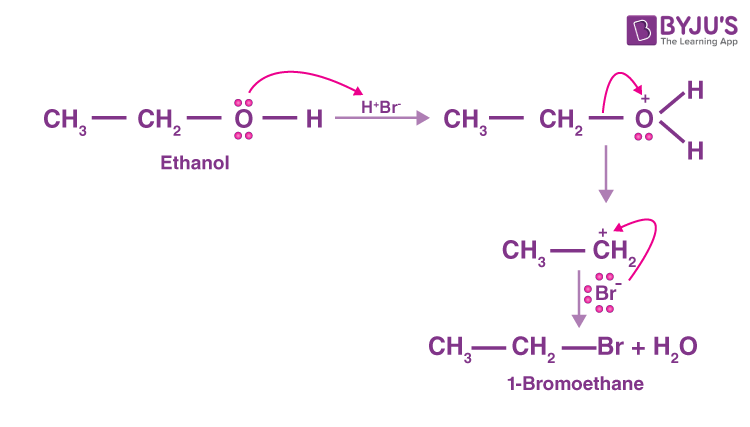Question 38:

Write the equations involved in the following reactions:

1. Reimer – Tiemann reaction

Reimer – Tiemann reaction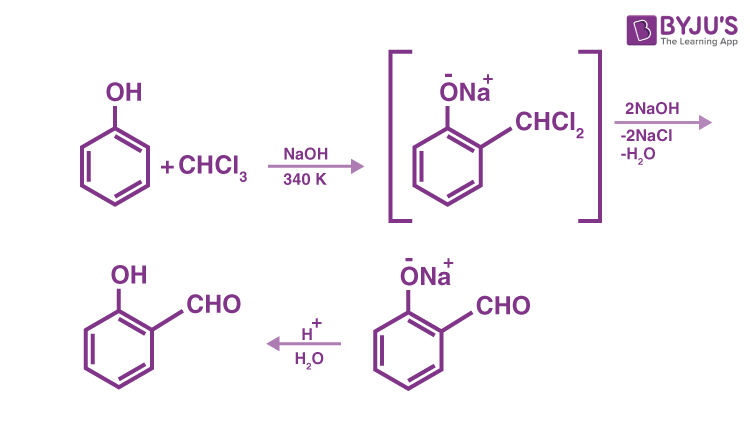1. Williamson synthesis

Williamson synthesis reaction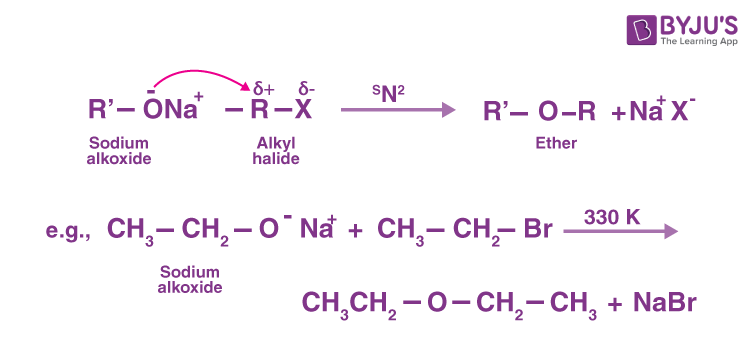Question 39:

Write the principle behind the froth floatation process. What is the role of collectors in this process?

The froth floatation process is based on the principle that metallic sulphide ore particles are preferentially wetted by oil while gangue particles are preferentially wetted by water.

Collectors attach themselves to mineral grains via polar groups, which become water repellent and thus pass into the froth. Ethyl xanthate and potassium ethyl xanthate are two examples.

Question 40:

For a chemical reaction R → P, the variation in the concentration (R) vs. time (t) plot is given as

(i) Predict the order of the reaction.

The variation in concentration (R) vs. time (t) plot shown here represents a zero-order reaction, in which the rate of the reaction is proportional to the zero power of the reactant concentration.

(ii) What is the slope of the curve?

The rate constant for a zero-order reaction is given as

[R] = [R]0 – kt

So, the slope of the curve for the variation in the concentration (R) vs. time (t) plot is equal to the negative of the reaction’s rate constant.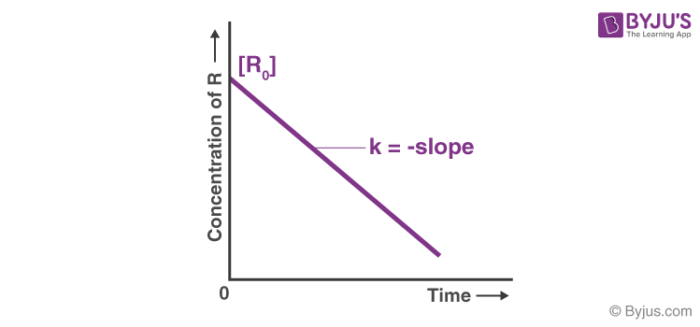Question 41:

(a) Why does the presence of an excess of lithium make LiCl crystals pink?

When there is an excess of Lithium in the form of a crystal of LiCl, the lithium atom loses an e to Li+ ions. The released e diffuses into the crystal and takes up residence in the anionic sites (F – centre). This e give the crystal its pink colour.

(b) A solid with cubic crystal is made of two elements P and Q. Atoms of Q are at the corners of the cube and P at the body centre. What is the formula of the compound?

It is given that the atoms of Q are present at the corners of the cube.

As a result, the number of Q atoms in one unit cell = 8 x 1/8 = 1.

It is also given that the P atoms are present at the body’s centre.

As a result, the number of P atoms in one unit cell equals one.

This means that the ratio of number of P atoms to the number of Q atoms, P:Q = 1:1.

As a result, the compound’s formula is PQ.

Question 42:

Define thermoplastic and thermosetting polymers. Give one example of each.

A thermoplastic polymer can be repeatedly softened and hardened by heating and cooling. As a result, it can be used repeatedly. Examples are polythene, polypropylene, etc.

A thermosetting polymer is a permanent setting polymer because it hardens and sets during the moulding process and cannot be re-softened. Examples are bakelite and melamine-formaldehyde polymers.

Question 43:

What is a biodegradable polymer? Give an example of a biodegradable aliphatic polyester.

Biodegradable polymers are those that degrade naturally over time due to environmental degradation by bacteria and other microorganisms. PHBV, i.e., poly −ꞵ− hydroxybutyrate – co – ꞵ − hydroxyvalerate is an example of a biodegradable aliphatic polyester.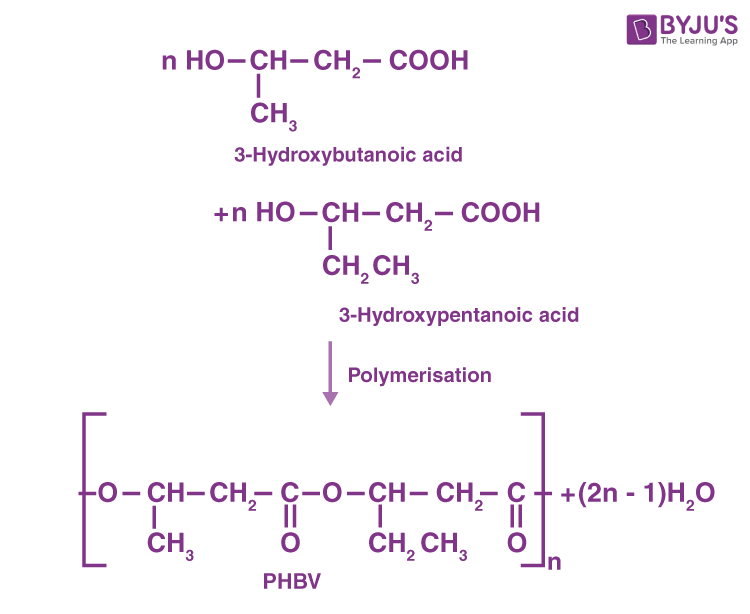Question 44:

Explain the mechanism of the following reaction CH3 – CH2 – OH

$$\begin{array}{l}\rightarrow[443 K]{H^+}\end{array}$$
CH2 = CH2 + H2O

Mechanism of acid catalysed dehydration of ethanol

Step I: Protonation of ethanol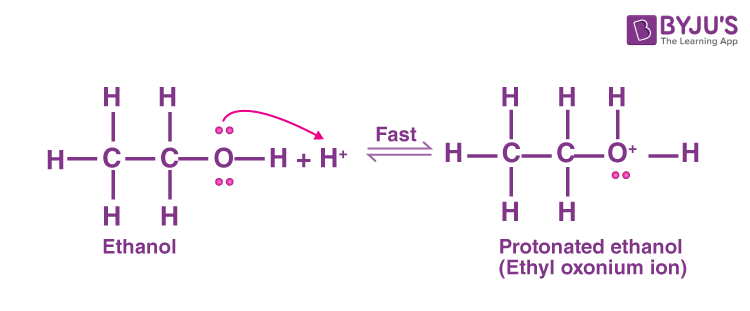Step II: Formation of the carbocation. It is the slowest step and rate-determining step.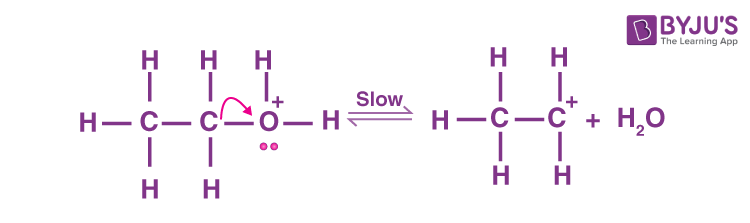Step III: Formation of ethene by elimination of a proton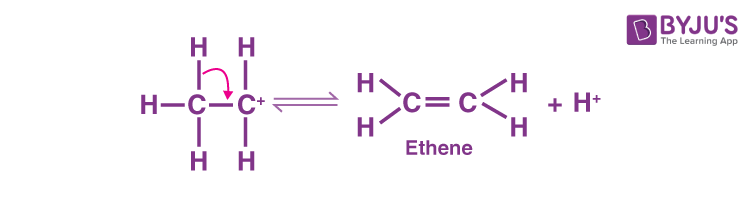Question 45:

How are interhalogen compounds formed? What can general compositions be assigned to them?

An inter-halogen compound is a molecule that contains two or more halogen atoms (fluorine, chlorine, bromine, iodine, or astatine) but no atoms from any other group.

The majority of known inter-halogen compounds are binary. Their formulae are typically XYn, where n is one of1,3,5, or 7, and X is the less electronegative of the two halogens. Inter-halogens are classified as follows:

• Diatomic interhalogens
• Tetratomic interhalogens
• Hexatomic interhalogens
• Octatomic interhalogens

Question 46:

Aluminium crystallizes in an fcc structure. The atomic radius of the metal is 125 pm. What is the length of the side of the unit cell of the metal?

For fcc structure, r = 125 pm,

$$\begin{array}{l}a=2\sqrt{2}r\end{array}$$

a= 2 ✕ 1.414 ✕ 1.25 pm = 353.5 pm ≅ 354 pm

So, the edge length of the metal is 354 pm.

Question 47:

The standard electrode potential (Eº) for Daniel cell is +1.1V. Calculate the ∆Gº for the reaction

Zn(s) + Cu2+(aq) → Zn2+ (aq) + Cu (s) (1F = 96500 C mol-1)

Zn (s) + Cu2+(aq) → Cu (s) + Zn2+(aq)

Therefore, the value of n=2

∆G°= -nFE°cell

∆G°= -2×96500 C mol-1×1.1 V

∆G°= – 212300 CV = -212300J [∵Coloumb × Volt = Joule]

∆G°= -212.3 KJ.

Question 48:

1. For a reaction A + B → P, the rate law is given by
r = k[A]1/2[B]2 What is the order of this reaction?

In the rate law, the overall order of a reaction is the sum of the exponents of the concentrations.

As a result, the order of the above reaction = 0.5 + 1 = 2.5.

1. A first-order reaction is found to have a rate constant k = 5.5 × 10-14 s–1. Find the half-life of the reaction.

A first-order reaction is found to have a rate constant, k= 5.5 ✕ 10–14 s–1

The following expression expresses the relationship between the half-life period (t1/2) and the rate constant, k, for a first-order reaction.

t1/2 = 0.693/k

t1/2 = 0.693/ 5.5 ✕ 10–14 s–1

t1/2 = 1.26 ✕ 10–13 s.

Question 49:

Outline the principles of refining of metals by the following methods:

(i) Zone refining

Zone refining: This method is based on the principle that impurities are more soluble in the metal’s melt than in its solid state.

(ii) Vapour phase refining